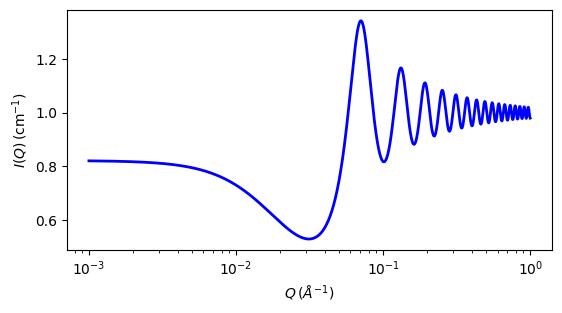# stickyhardsphere

‘Sticky’ hard sphere structure factor with Percus-Yevick closure

Parameter

Description

Units

Default value

50

volfraction

volume fraction of hard spheres

None

0.2

perturb

perturbation parameter, tau

None

0.05

stickiness

stickiness, epsilon

None

0.2

The returned value is a dimensionless structure factor, $$S(q)$$.

Calculates the interparticle structure factor for a hard sphere fluid with a narrow, attractive, potential well. Unlike the squarewell model, here a perturbative solution of the Percus-Yevick closure relationship is used. The strength of the attractive well is described in terms of “stickiness” as defined below.

The perturbation parameter (perturb), $$\tau$$, should be fixed between 0.01 and 0.1 and the “stickiness”, $$\epsilon$$, allowed to vary to adjust the interaction strength. The “stickiness” is defined in the equation below and is a function of both the perturbation parameter and the interaction strength. $$\epsilon$$ and $$\tau$$ are defined in terms of the hard sphere diameter $$(\sigma = 2 R)$$, the width of the square well, $$\Delta$$ (having the same units as $$R$$), and the depth of the well, $$U_o$$, in units of $$kT$$. From the definition, it is clear that smaller $$\epsilon$$ means a stronger attraction.

$\begin{split}\epsilon &= \frac{1}{12\tau} \exp(u_o / kT) \\ \tau &= \Delta / (\sigma + \Delta)\end{split}$

where the interaction potential is

$\begin{split}U(r) = \begin{cases} \infty & r < \sigma \\ -U_o & \sigma \leq r \leq \sigma + \Delta \\ 0 & r > \sigma + \Delta \end{cases}\end{split}$

The Percus-Yevick (PY) closure is used for this calculation, and is an adequate closure for an attractive interparticle potential. The solution has been compared to Monte Carlo simulations for a square well fluid, with good agreement.

The true particle volume fraction, $$\phi$$, is not equal to $$h$$ which appears in most of reference . The two are related in equation (24). Reference  also describes the relationship between this perturbative solution and the original sticky hard sphere (or “adhesive sphere”) model of Baxter .

Note

The calculation can go haywire for certain combinations of the input parameters, producing unphysical solutions. In this case errors are reported to the command window and $$S(q)$$ is set to -1 (so it will disappear on a log-log plot!).

Use tight bounds to keep the parameters to values that you know are physical (test them), and keep nudging them until the optimization does not hit the constraints.

Note

Earlier versions of SasView did not incorporate the so-called $$\beta(q)$$ (“beta”) correction  for polydispersity and non-sphericity. This is only available in SasView versions 5.0 and higher.

In SasView the effective radius may be calculated from the parameters used in the form factor $$P(q)$$ that this $$S(q)$$ is combined with.

For 2D data the scattering intensity is calculated in the same way as 1D, where the $$q$$ vector is defined as

$q = \sqrt{q_x^2 + q_y^2}$Fig. 129 1D plot corresponding to the default parameters of the model.

Source

stickyhardsphere.py

References

1. S V G Menon, C Manohar, and K S Rao, J. Chem. Phys., 95(12) (1991) 9186-9190

2. R J Baxter, J. Chem. Phys., 49 (1968), 2770-2774

3. M Kotlarchyk and S-H Chen, J. Chem. Phys., 79 (1983) 2461-2469

Authorship and Verification

• Author: